# Enumeration:-------------------------------
An enumeration is a complete, ordered listing of all the items in a collection. The term is
commonly used in mathematics and computer science to refer to a listing of all of the elements of a
set. The precise requirements for an enumeration depend on the discipline of study and the context
of a given problem. <https://en.wikipedia.org/wiki/Enumeration>
-------------------------------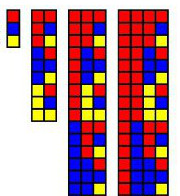## Notes on the "Base Enumeration" Page: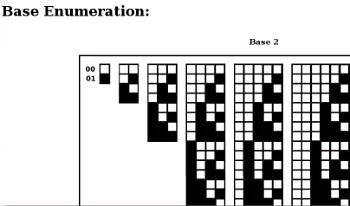### Base (mathematics)

In mathematics, a base or radix is the number of different digits
or combination of digits and letters that a system of counting uses to represent numbers.
For example, the most common base used today is the decimal system.
Because "dec" means 10, it uses the 10 digits from 0 to 9.
Most people think that we most often use base 10 because we have 10 fingers.

A base can be any whole number bigger than 1.
The base of a number may be written next to the number: for instance,
${\displaystyle 23_{8}}$238 means 23 in base 8 (which is equal to 19 in base 10).

-------

### Base 2: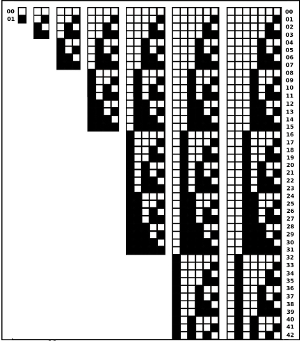Base 2 is represented by 1 to 7 numerical places
Base 3: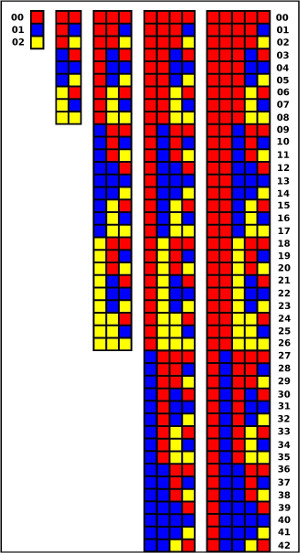Base 3 is represented by 1 to 5 numerical places
Base 4: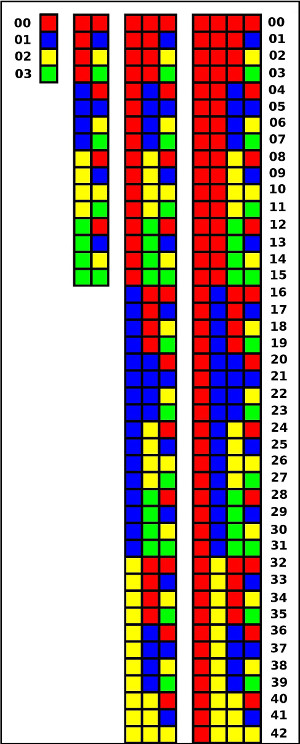Base 4 is represented by 1 to 4 numerical places
Combined Bases 1: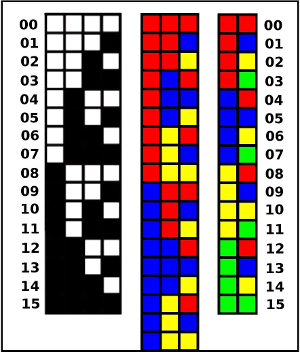The first 15 numbers are represented in bases 2 - 4
Combined Bases 2:The Base 2, 3, and 4 images are combined -for comparison

-------------------------------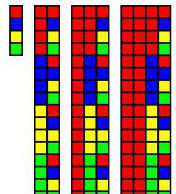-------------------

Number Spaces

Counting
-------------------

Trevor Batten
<trevor at tebatt dot net>
Baclayon 2019
home